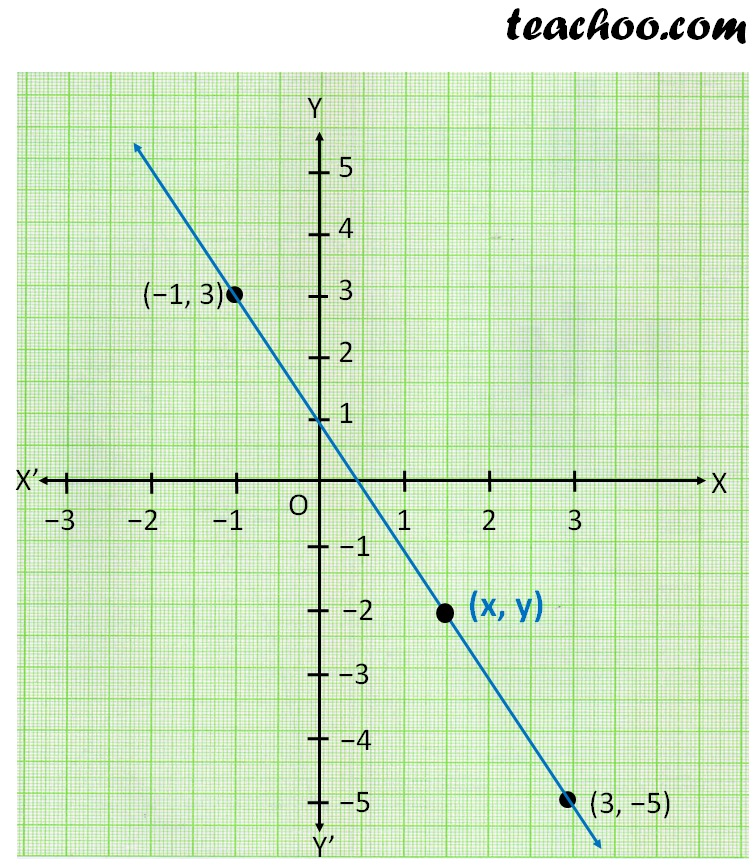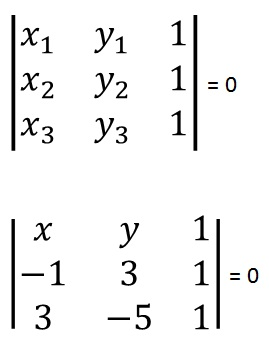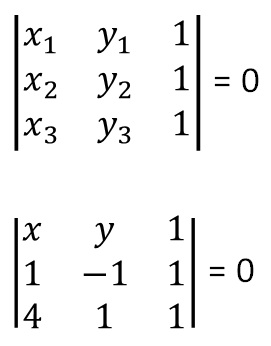Equation of line using determinant

Chapter 4 Class 12 Determinants
Concept wise

Let’s suppose we need to find

equation of line passing through

(−1, 3), (3, −5)

If we try to draw the graph, we see thatNow,

Let’s take any point (x, y) on the line

We see that (x, y), (−1, 3), (3, −5) are on the same line

So, their Area of triangle = 0

Area of triangle = 0x (3 × 1 − (−5) × 1) − y ((−1) × 1 − 3 × 1) + 1 ((−1) × (−5) − 3 × 3) = 0

x (3 + 5) − y (−1 − 3) + (5 − 9) = 0

8x + 4y − 4 = 0

4 (2x + y − 1) = 0

2x + y − 1 = 0

So, equation of line is 2 x + y − 1 = 0

## Find equation of line passing through (1, −1) & (4, 1), using determinants

Let (x, y) be a point on the required line

So, (x, y), (1, −1) & (4, 1) are in a same line

Therefore,

Area of triangle formed by them = 0𝑥 ((−1) × 1 − 1) − y (1 × 1 − 4 × 1) + 1 (1 × 1 −4 × (−1)) = 0

𝑥 (−1 − 1) − y (1 − 4) + 1 (1 + 4) = 0

−2𝑥 + 3y + 5 = 0

3y − 2𝑥 + 5 = 0

Thus, the required condition of the line is 3y − 2𝒙 + 5 = 0

Learn in your speed, with individual attention - Teachoo Maths 1-on-1 Class

### Transcript

|■8(𝑥_1&𝑦_1&1@𝑥_2&𝑦_2&1@𝑥_3&𝑦_3&1)| = 0 |■8(𝑥&𝑦&1@−1&3&1@3&−5&1)| = 0 |■8(𝑥_1&𝑦_1&1@𝑥_2&𝑦_2&1@𝑥_3&𝑦_3&1)| = 0 |■8(𝑥&𝑦&1@1&−1&1@4&1&1)| = 0